Next: Jacobi Integral Up: The Three-Body Problem Previous: Introduction

# Circular Restricted Three-Body Problem

Consider an isolated dynamical system consisting of three gravitationally interacting point masses,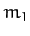,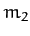, and. Suppose, however, that the third mass,, is so much smaller than the other two that it has a negligible effect on their motion. Suppose, further, that the first two masses,and, execute circular orbits about their common center of mass. In the following, we shall investigate this simplified problem, which is generally known as the circular restricted three-body problem.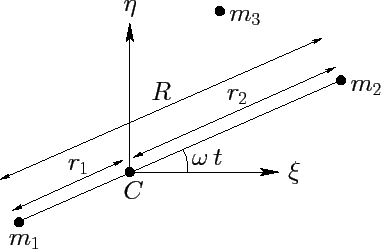Let us define a Cartesian coordinate system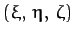in an inertial reference frame whose origin coincides with the center of mass,, of the two orbiting masses. Furthermore, let the orbital plane of these masses coincide with the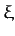-plane, and let them both lie on the-axis at time--see Figure 47. Suppose thatis the constant distance between the two orbiting masses,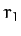the constant distance between massand the origin, and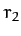the constant distance between massand the origin. Moreover, let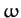be the constant orbital angular velocity. It follows, from Section 6.3, that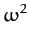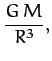(1030)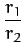(1031)

where.

It is convenient to choose our unit of length such that, and our unit of mass such that. It follows, from Equation (1030), that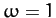. However, we shall continue to retainin our equations, for the sake of clarity. Let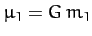, and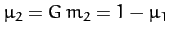. It is easily demonstrated that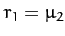, and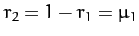. Hence, the two orbiting masses,and, have position vectors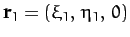and, respectively, where (see Figure 47)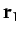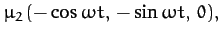(1032)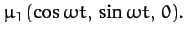(1033)

Let the third mass have position vector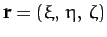. The Cartesian components of the equation of motion of this mass are thus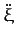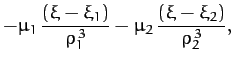(1034)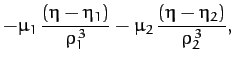(1035)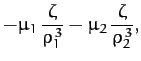(1036)

where(1037)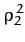(1038)Next: Jacobi Integral Up: The Three-Body Problem Previous: Introduction
Richard Fitzpatrick 2011-03-31